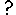# Conditional submodels

A conditional submodel is used when there are conditions under which a submodel, or an instance of a fixed-membership multiple-instance submodel, may or may not exist. By "exist" we mean that Simile evaluates the expressions in the submodel. The existence of each instance of the submodel is determined by evaluating a Boolean expression in acondition symbol placed inside the submodel.

The conditional submodel may represent a phenomenon that only occurs at certain times, for instance bush fires. Or it may represent a phenomenon that occurs in some, but not all, of the members of a group; for instance, if an area of interested is represented by a grid, then some vegetation types, e.g., forest, may be present in only some of the grid squares, so the processes associated with them sould be represented in a conditional submodel of the grid-square model.

If the submodel has influence arrows going from inside to variables outside itself, then:

• if the submodel exists, the values emerging from the submodel are those calculated from the variables in the normal way;
• if the submodel (or instance of it) does not exist, then a value is not exported for each variable: there is no value, since the variable just doesn't exist.

This means that we cannot be sure how many values are going to be exported from a single variable inside the conditional submodel. For a simple submodel, there could be zero or one values. For a fixed-membership multiple-instance submodel, there could be 0…n values, where n is the number of instances for the submodel.

Therefore values are always exported as a list from a conditional model, since a list (as opposed to an array) is the data structure used when the number of values in the data structure can vary dynamically over time. A list must be processed as soon as it is received: it cannot be passed around as a persistent data structure. Typically, you will simply use the sum function to add the values in the list together.

A few more detailed considerations:

• The condition equation itself, and the equations of any variables in the submodel that affect the condition, must be evaluated in every case. If the condition comes out false, the values of these variables are discarded.
• The condition cannot depend on a value outside the submodel that itself depends directly on values inside, since getting this value would require the existence status of the submodel to be already known. Simile reports this as a circularity problem.